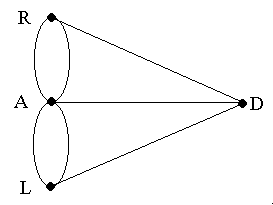# Euler Graph - 1736 Seven Bridges of Königsberg Problem

Given the graph below, is it possible to construct a path (or a cycle, i.e. a path starting and ending on the same vertex) which visits each edge exactly once ?

All vertices in a graph for which an Euler circuit exists must have even degree.The first thing that Euler did was boil the Konigsberg problem down to its essentials by stripping away all irrelevant material:

What results is an abstract representation of Konigsburg that depicts just enough (and not a whit more) about the problem to completely describe it.  This simplified form of the problem makes it easier to study as a mathematical object, and to devise some mathematical theorems about it.  Which is exactly what Euler did.
The sum of the degrees of all vertices in any graph is twice the number of edges.